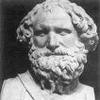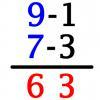#### You may also like### Archimedes and Numerical Roots

The problem is how did Archimedes calculate the lengths of the sides of the polygons which needed him to be able to calculate square roots?### Triangle Incircle Iteration

Keep constructing triangles in the incircle of the previous triangle. What happens?### Vedic Sutra - All from 9 and Last from 10

Vedic Sutra is one of many ancient Indian sutras which involves a cross subtraction method. Can you give a good explanation of WHY it works?

# Zeller's Birthday

### Why do this problem?

This problem introduces students to the idea of an algorithm. We hope that the context of finding the day of the week for key dates adds a little extra interest. Beneath it all is the important idea of modulo arithmetic.

### Possible approach

Start with some dates for which students feel confident they know the day of the week. It might be a recent date (Christmas last year, or a recent wedding) or something like a major sporting event which happens on a particular day (often Saturday).

Ask if any student happens to know the day in the week on which they were born. Students might ask their parent or guardian in preparation for this lesson.

Then invite students to try out the algorithm with some birth dates.

Check that the method is understood and practised to the point of familiarity.

That brings the group to the heart of the problem : how does this algorithm do its job ?

One way to explore that question is to think about the procedure we would need to follow if this was a 'one off' problem and for which we had no ready-made algorithm available.

### Key questions

• How does this algorithm do its job ?
• Why 2.6 and why 5.39 ?
• How would you find the day of the week on which you were born if there was no ready-made algorithm available ?

### Possible extension

Design a spreadsheet that uses the algorithm to report the day of the week for any given birth date.

### Possible support

Students could be introduced to the idea of algorithms using a more familiar context, such as the long multiplication algorithms in this problem.